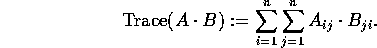Trace of a Product MatrixNext: Run Time Output Up: C-XSC Sample Programs Previous: Run Time Output

## Trace of a Product Matrix

Dot product expressions are sums of real, interval, complex, or cinterval constants, variables, vectors, matrices, or simple products of them. Dotprecision variables are used to store intermediate results of a dot product expression without any rounding error. The contents of a dotprecision variable may be rounded into a floating-point number using the rounding direction specified by the user.

The following C-XSC program demonstrates the use of this concept. The trace of a complex matrix AB is evaluated without calculating the actual product. The result is of maximum accuracy. That is, it is the best possible approximation of the exact result. The trace of the product matrix is```#include "cmatrix.hpp"     // Use the complex matrix package
main()
{
int n;
cout << "Please enter the matrix dimension n: ";  cin  >> n;
cmatrix A(n,n), B(n,n);  // Dynamic allocation of A, B
complex result;  cdotprecision accu;
cout << "Please enter the matrix A:" << endl;  cin  >> A;
cout << "Please enter the matrix B:" << endl;  cin  >> B;
accu = 0.0;              // Clear accumulator
for (int i=1;	i<=n; i++)  accumulate(accu, A[i], B[Col(i)]);
// A[i] and B[Col(i)] are subarrays of type cvector.
// Rounding the exact result to the nearest complex number:
result = rnd(accu);
cout << SetPrecision(15,8) << RndNext << Dec;
cout << "The trace of the product matrix is: " << endl << result << endl;
}```

Andreas Wiethoff
Wed Mar 29 13:50:24 MET DST 1995